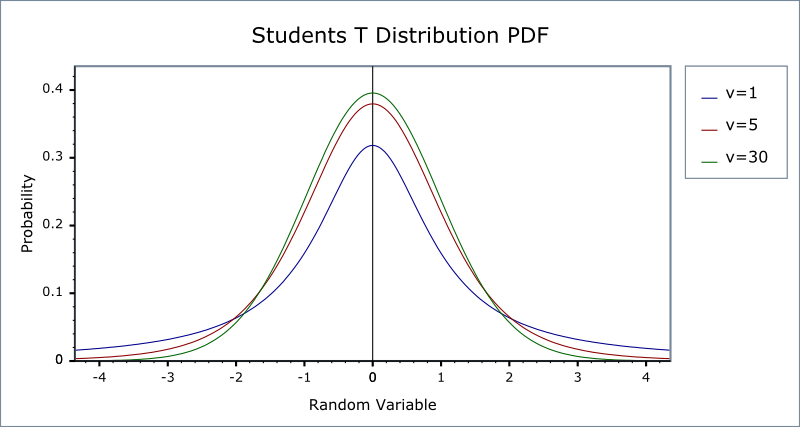#Boost C++ Libraries

...one of the most highly regarded and expertly designed C++ library projects in the world.

This is the documentation for an old version of boost. Click here for the latest Boost documentation.
##### Students t Distribution

`#include <boost/math/distributions/students_t.hpp>`

```namespace boost{ namespace math{

template <class RealType = double,
class Policy   = policies::policy<> >
class students_t_distribution;

typedef students_t_distribution<> students_t;

template <class RealType, class Policy>
class students_t_distribution
{
typedef RealType value_type;
typedef Policy   policy_type;

// Construct:
students_t_distribution(const RealType& v);

// Accessor:
RealType degrees_of_freedom()const;

// degrees of freedom estimation:
static RealType find_degrees_of_freedom(
RealType difference_from_mean,
RealType alpha,
RealType beta,
RealType sd,
RealType hint = 100);
};

}} // namespaces
```

A statistical distribution published by William Gosset in 1908. His employer, Guinness Breweries, required him to publish under a pseudonym, so he chose "Student". Given N independent measurements, letwhere M is the population mean,μ is the sample mean, and s is the sample variance.

Student's t-distribution is defined as the distribution of the random variable t which is - very loosely - the "best" that we can do not knowing the true standard deviation of the sample. It has the PDF:The Student's t-distribution takes a single parameter: the number of degrees of freedom of the sample. When the degrees of freedom is one then this distribution is the same as the Cauchy-distribution. As the number of degrees of freedom tends towards infinity, then this distribution approaches the normal-distribution. The following graph illustrates how the PDF varies with the degrees of freedom ν:##### Member Functions
```students_t_distribution(const RealType& v);
```

Constructs a Student's t-distribution with v degrees of freedom.

Requires v > 0, otherwise calls domain_error. Note that non-integral degrees of freedom are supported, and meaningful under certain circumstances.

```RealType degrees_of_freedom()const;
```

Returns the number of degrees of freedom of this distribution.

```static RealType find_degrees_of_freedom(
RealType difference_from_mean,
RealType alpha,
RealType beta,
RealType sd,
RealType hint = 100);
```

Returns the number of degrees of freedom required to observe a significant result in the Student's t test when the mean differs from the "true" mean by difference_from_mean.

difference_from_mean

The difference between the true mean and the sample mean that we wish to show is significant.

alpha

The maximum acceptable probability of rejecting the null hypothesis when it is in fact true.

beta

The maximum acceptable probability of failing to reject the null hypothesis when it is in fact false.

sd

The sample standard deviation.

hint

A hint for the location to start looking for the result, a good choice for this would be the sample size of a previous borderline Student's t test.

NoteRemember that for a two-sided test, you must divide alpha by two before calling this function.

For more information on this function see the NIST Engineering Statistics Handbook.

##### Non-member Accessors

All the usual non-member accessor functions that are generic to all distributions are supported: Cumulative Distribution Function, Probability Density Function, Quantile, Hazard Function, Cumulative Hazard Function, mean, median, mode, variance, standard deviation, skewness, kurtosis, kurtosis_excess, range and support.

The domain of the random variable is [-∞, +∞].

##### Examples

Various worked examples are available illustrating the use of the Student's t distribution.

##### Accuracy

The normal distribution is implemented in terms of the incomplete beta function and it's inverses, refer to accuracy data on those functions for more information.

##### Implementation

In the following table v is the degrees of freedom of the distribution, t is the random variate, p is the probability and q = 1-p.

Function

Implementation Notes

pdf

Using the relation: pdf = (v / (v + t2))(1+v)/2 / (sqrt(v) * beta(v/2, 0.5))

cdf

Using the relations:

p = 1 - z iff t > 0

p = z otherwise

where z is given by:

ibeta(v / 2, 0.5, v / (v + t2)) / 2 iff v < 2t2

ibetac(0.5, v / 2, t2 / (v + t2) / 2 otherwise

cdf complement

Using the relation: q = cdf(-t)

quantile

Using the relation: t = sign(p - 0.5) * sqrt(v * y / x)

where:

x = ibeta_inv(v / 2, 0.5, 2 * min(p, q))

y = 1 - x

The quantities x and y are both returned by ibeta_inv without the subtraction implied above.

quantile from the complement

Using the relation: t = -quantile(q)

mean

0

variance

v / (v - 2)

mode

0

skewness

0

kurtosis

3 * (v - 2) / (v - 4)

kurtosis excess

6 / (df - 4)

 Copyright © 2006 , 2007, 2008 John Maddock, Paul A. Bristow, Hubert Holin, Xiaogang Zhang and Bruno Lalande Distributed under the Boost Software License, Version 1.0. (See accompanying file LICENSE_1_0.txt or copy at http://www.boost.org/LICENSE_1_0.txt)